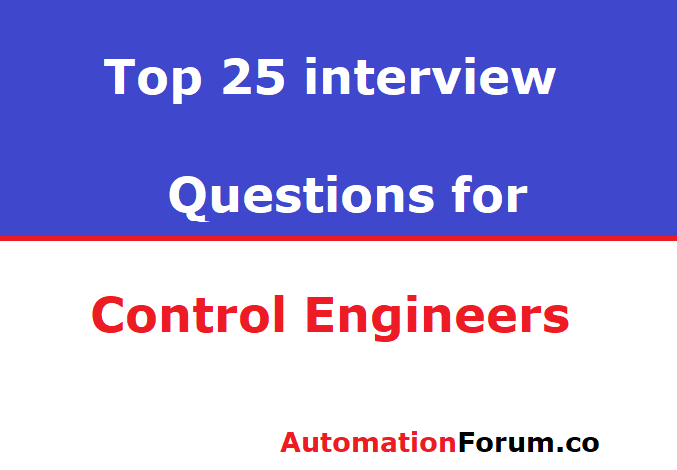# Top 25 Interview Questions for Control engineers### 1. What is a control system?

The control system the output and inputs are interrelated in such a manner that the output quantity or variable is controlled by input quantity, then such a system is called control system.

### 2. What are the different types of control systems?

• Open Loop control system: The open loop control system is one where the amount of output does not affect the amount of input. There is no feedback from output to input.
• Closed Loop control system: The closed loop control system provides feedback from the correction output amount. so as to maintain the desired output of the system

### 3. What is feedback in control system?

The control system feedback in one where the output is sampled and the proportional signal is returned to the input for automatic error correction for further processing to return the required output

### 4. What is the effect of positive feedback on the stability of the system?

Positive feedback is usually not used in the control system as it improves instability in the error signal and drive scheme. In minor loop control schemes, however, positive feedback is used to amplify certain inner signals and parameters

### 5. What is the frequency response?

A frequency response is a system’s steady reaction when the system input is a sinusoidal signal

### 6. List out the different frequency domain specifications?

• Resonant peak: The maximum value of the magnitude of closed-loop transfer function is called resonant peak.
• Resonant frequency: The frequency at which resonant peak occurs is called resonant frequency.

### 7. What is Bandwidth?

The bandwidth is the frequency range for which the system gain is more the 3db. The bandwidth is a measure of the feedback system’s capacity to reproduce the input signal, the features of noise rejection, and rises time.

### 8. Define Cut-off rate?

The cutoff rate is called the slope of the log magnitude curve near the cutoff. The cut-off frequency shows the capacity to distinguish between the signal and the noise

### 9. Define Gain margin?

The gain margin is defined as the reciprocal of the magnitude of the open loop transfer function at phase cross over frequency.

### 10. What is phase margin?

The phase margin is the amount of phase lag at the gain cross over frequency required to bring system to the verge of instability.

### 11. What is Bode plot?

The bode plot is the system’s transfer fucntion frequency response plot. There are two graphs in a bode plot. One is the plot of magnituse of sinusoidal transfer function VS logw. The other is a plot of the sinusoidal function stage angle

### 12.What is Nichols chart?

The chart consisting if M & N loci in the log magnitude versus phase diagram is called Nichols chart.

### 13. What is a compensator?

A device inserted into the system for the purpose of satisfying the specifications is called as a compensator.

### 14. What is a signal flow graph?

A signal flow diagram is a diagram representing a collection of algebraic equations simultaneously. By taking LT the time domain differential equations governing a control system can be transferred to set of the algebraic equation in s-domain.

### 15. What is sink and source?

Source is the input node in the signal flow graph and only branches are outgoing. Sinking is an output node in the signal flow graph and has only branches coming in

### 16. What is transmittance?

The transmittance is the gain the signal acquires when it moves in the signal flow graph from one node to another.

### 17. What is servomechanism?

The servomechanism is a feedback control system in which the output is mechanical position.

### 18. What is order of a system?

The order of a model is the order of the system regulating differential equation. The system order can be acquired from a specified system’s transfer function.

### 20. Why the derivative controller is not used in control systems?

The derivative controller produces control measures depending on the rate of change in the error signal and produces no corrective steps for any constant error.

### 21. What is the disadvantage in the proportional controller?

It produces a constant steady state error.

### 22. Name the test signals used in control system:

Test input control system are impulse step ramp acceleration and sinusoidal signals.

### 23. What is the necessary condition for stability?

All the coefficient of the characteristic polynomial be positive.

### 24. What is the mathematical model of a control system?

Control system is the set of connected physical components to serve an goal. Differential equations govern the output and input relationship of different physical systems. A control system’s mathematical model is a collection of different equations.

### 25. What is Time response of the control system?

The control system’s time response is described as a function of time as the output of the closed loop system. The system’s time response can be acquired by solving the system’s differential equation or time response

Scroll to Top